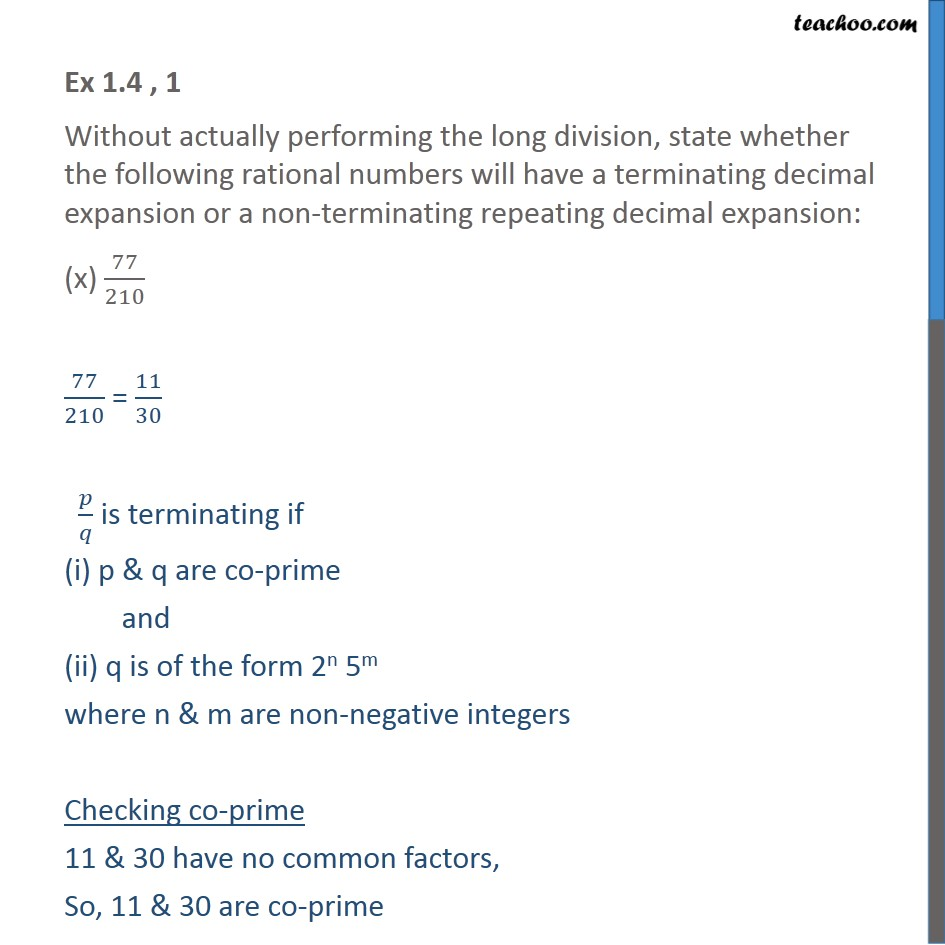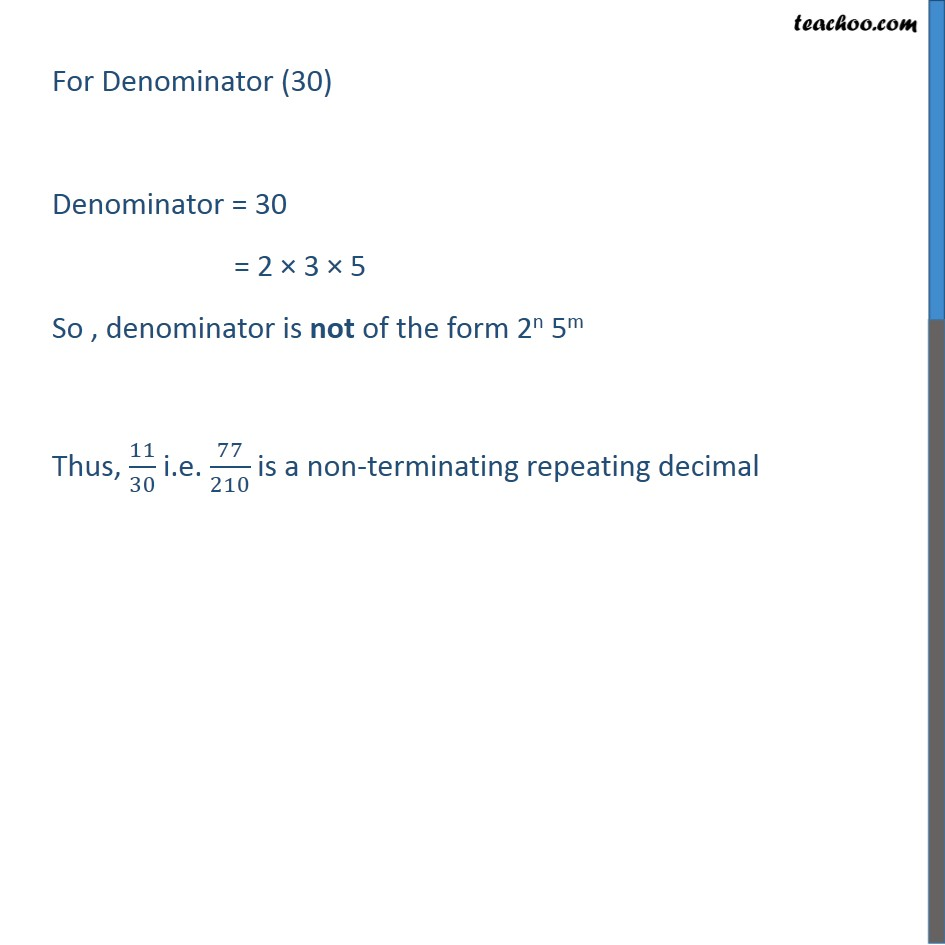1. Chapter 1 Class 10 Real Numbers (Term 1)
2. Serial order wise
3. Ex 1.4

Transcript

Ex 1.4 , 1 Without actually performing the long division, state whether the following rational numbers will have a terminating decimal expansion or a non-terminating repeating decimal expansion: (x) 77/210 77/210 = 11/30 𝑝/𝑞 is terminating if (i) p & q are co-prime and (ii) q is of the form 2n 5m where n & m are non-negative integers Checking co-prime 11 & 30 have no common factors, So, 11 & 30 are co-prime For Denominator (30) Denominator = 30 = 2 × 3 × 5 So , denominator is not of the form 2n 5m Thus, 11/30 i.e. 77/210 is a non-terminating repeating decimal

Ex 1.4